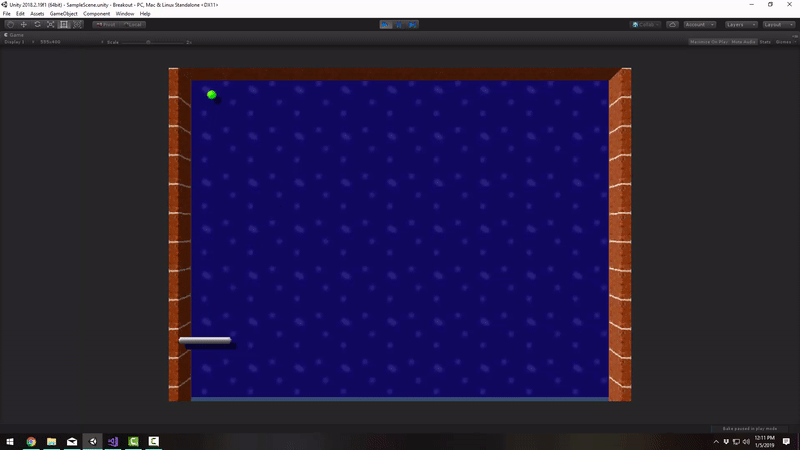Hello. I am working on a Breakout-style Unity game and I am having an issue the interaction between the ball and the paddle. I want to make it so that depending on where the ball hits the edges of the paddle it will angle out more along the x axis. The way my code is currently working the balle always keeps the same angle no matter where it hits the paddle. Here is my code for the ball bouncing off the paddle. Thanks for any help!

``````if (collider.gameObject.name == "Paddle")
{
float x = hitFactor(transform.position, collider.transform.position, collider.collider.bounds.size.x);
Vector2 dir = new Vector2(x, 1).normalized;
GetComponent<Rigidbody2D>().velocity = dir * ballSpeed * Time.deltaTime;
}

{
}
``````This is how I did it in my own breakout clone and it seemed to do the trick.

[UPDATE] I’ve replaced the coroutine with an invoke statement, and replaced the ‘if statements’ inside OnCollisionEnter2D with a cleaner switch statement. The code still runs as expected.

``````using UnityEngine;

public class Ball : MonoBehaviour
{
[SerializeField]
private float _speed;

private Rigidbody2D _rb;

private Vector2 _direction;

private void Start()
{
_rb = GetComponent<Rigidbody2D>();
Invoke("DropBall", 1);
}

private void DropBall()
{
int random = Random.Range(-1, 2);
_direction = new Vector2(random, -1).normalized;
}

private void FixedUpdate()
{
_rb.velocity = _direction * _speed;
}

private void OnCollisionEnter2D(Collision2D other)
{
switch (other.gameObject.tag)
{
{
float x = HitFactor(transform.position, other.transform.position, other.collider.bounds.size.x);
_direction = new Vector2(x, 1).normalized;
break;
}
case "SideWall":
{
_direction.x *= -1;
break;
}
case "Ceiling":
{
_direction.y *= -1;
break;
}
}
}